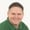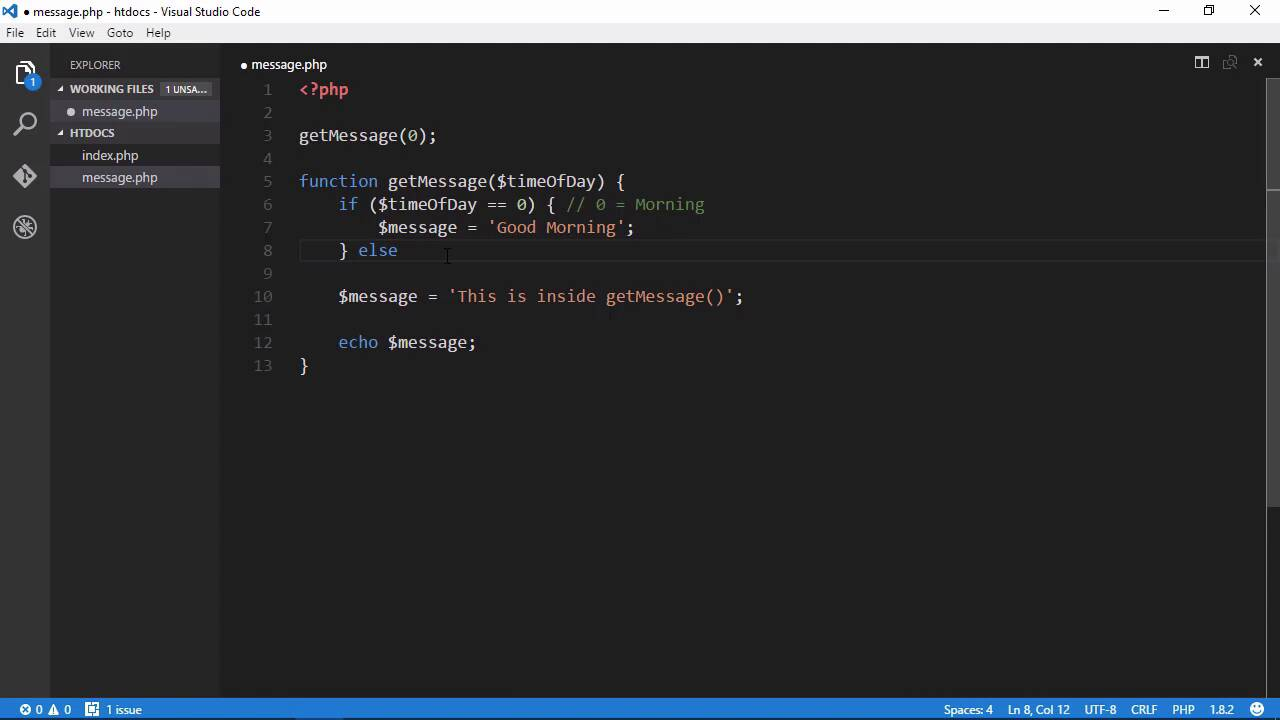Lessons: 33Length: 4.8 hours• Overview
• Transcript

# 8.4 Parameters and Decisions

Our code is beginning to take shape, but our function doesn't do much yet. Let's make it process some data. To do that, we'll add a parameter to the function and make decisions based upon the value of that parameter.

## 11.Conclusion1 lesson, 01:47

### 8.4 Parameters and Decisions

In the previous lesson, we created a variable, and we also created a function. And all that function does right now is display a message. Wouldn't it be cool though if we changed the function, so that depending upon the timeOfDay, it would display a different message? So in order for that to occur, the getMessage function needs to know what timeOfDay it is. And the best way to do that is to pass a value to that function that denotes a certain timeOfDay. Now, we would do that by simply passing a value to the function, whatever we call it. So we will have getMessage, followed by an open parentheses, and then the value. So let's say that 0 would be morning. So if we pass 0 to getMessage, then that would denote morning. So the getMessage function would then display a message that says something like good morning. Now, in order to make that work, the getMessage function needs to denote that it accepts a value. And we do that by adding a parameter in between the opening and closing parentheses. So this is going to represent the timeOfDay. So let's just call it timeOfDay. And a parameter looks a lot like a variable. In fact, for all intents and purposes, it is a variable. So inside of this function, we can use this timeOfDay variable, just like we would this message variable. So now that we know what timeOfDay it is, we need to decide what message to display, based upon what timeOfDay it is. To do that, we need to make a decision. And decisions are made with a special keyword called if. So basically, we're going to say if timeOfDay is equal to 0, which means morning, then we want to display a certain message. So in code, it starts with if. And then we have what's called the condition. The condition is something that is going to result in either true or false. So our condition, in this case, is going to be if {;$timeOfDay == 0}. We are comparing timeOfDay to the value of 0. And notice that we were doing so using two equal signs. We aren't using a single equals sign, because what that means is we would be assigning the value of 0 to timeOfDay. And that is not what we want to do, we want to compare timeOfDay with 0. So we have two equal signs there. And then we have an opening and closing curly brace, so that if this condition is true, then the code inside of these curly braces is going to execute. So in this case, we can say that our message = good morning. And that's all that we would need to do there. Now, this is a little cryptic, because we have the value of 0, and we don't really know what that value means if we were just looking at the code. Or rather, if somebody else is looking at our code. So what we're going to do is add a comment. It's really just some text that is for our purposes, so that we can explain our code. It's not there for PHP. In fact, PHP is going to ignore our comment. But here, we can say that 0 = morning. And that way, whenever we come back and revisit this code at a later time, or somebody else is looking at our code, they can say that okay, we are seeing that if this is morning. Now, ideally, we want to avoid comments as much as possible, because that basically means that our code isn't very readable. But in this case, it's a good idea, just so that we know that 0 is morning. Now, if the timeOfDay is not equal to 0, then we want to do something else. And we do that with a keyword called else. After the closing curly brace of the if code block, we have else, and then we have another code block. So we have a code block for the if statement that executes if the condition is true. Then we have an else block that will execute if the condition is false. So if {$timeOfDay == 1}, then we could say that it's afternoon. So then our message could be good afternoon. But there are more times of day than just morning and afternoon. There's evening, and then some people consider night to be the final part of the day. So in this case, let's say that we also want to check to see if it's evening as well. Well, we can't really do that here, because we have a check to see if the timeOfDay is 0. And if that's not true, then we are automatically just saying that okay, then it's afternoon. Now, inside of this else block, we could have another if statement. And we could say that if ($timeofday == 1), then we would display good afternoon. And then we could have another else block there, and that could be used for evening, but there's a better way to do that. So let's get rid of that. And instead of saying just else here, we're going to say else if, so that we can test for another condition. And all we have to do is just write that other condition. If {$timeOfDay == 1}, then we have the afternoon. So then we can say that our message is equal to good afternoon. Let's go ahead and add a comment that says that 1 is equal to afternoon. And then we could have an else statement. But we could also add yet another else if. So if we wanted to say that if {\$timeOfDay == 3}, then that would be nighttime, and we could add good night, but let's not do that. And so we're just going to have a normal else statement, and we are going to set message = good evening. So there are three possible outcomes of executing getMessage. If the timeOfDay is 0, then we will have good morning. If timeOfDay is 1, then we will have good afternoon. Otherwise, we will have good evening. So let's go ahead and execute this. We're already passing 0 to getMessage. So let's go to the browser, refresh the page, and we see good morning. So let's pass the value of 1 to getMessage, and we should see good afternoon. But we don't we see good evening, so something is wrong with our code, because that is not what is supposed to happen. So we need to look at this, and the else if is fine. The timeOfDay == 1. And I see the problem. We have a lowercase D here for timeOfDay. And this is a very good lesson, because PHP is case sensitive. There was a difference between timeOfDay with a capital D, and timeOfDay with a lowercase d. As far as PHP is concerned, they are two different variables. So because timeOfDay was not 0, and because timeOfDay with a lowercase d is not 1, because we didn't create that. We didn't assign it a value, so that is obviously false. It defaulted to good evening. So we need to change this to an uppercase D. Let's go back to the browser, and now we should see good afternoon, and then we can pass anything other than 0 or 1, and we should get evening. Let's just do the value of 2, and we will see good evening. So now we have a function that is processing data. It is accepting data and doing something with that by displaying a message based upon the timeOfDay. The only thing we need to do now is let the user choose what timeOfDay it is, so that we can get that information from the user, and then display our message. And we will do that in the next lesson.

Back to the top